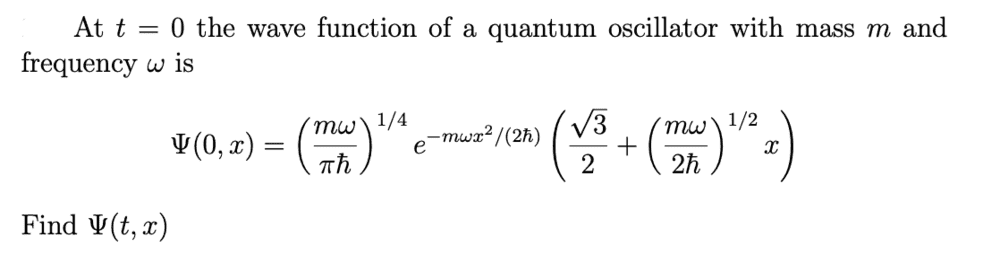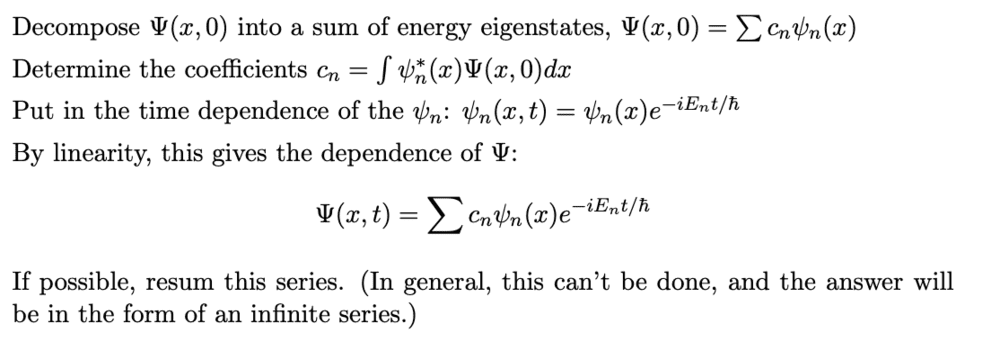# Find the time-dependent wave function Ψ(x, t).

• gabriellelee

#### gabriellelee

Homework Statement
Find the time-dependent wave function Ψ(x, t).
Relevant Equations
Please see below for the full question.I thought I could start somewhere along the lines of ##\psi(x,t)= \psi(x,0)e^{-iE_nt/\hbar}##, but I'm not sure what ##E_n## would be.
I also thought about doing the steps listed below in the picture, but I'm not sure how to decompose ##\psi(x,0)## like it says to in the first step.
Any help would be very much appreciated.This is the standard method for solving the Schroedinger equation. In this case the decomposition into energy eigenstates has been done for you! You need to look more closely at the initial wavefunction:

Do you recognise anything there?

Are the right two terms ##\psi(x)## and the first term is ##C_n##?

Are the right two terms ##\psi(x)## and the first term is ##C_n##?
No.

Look at the explicit formulation of the first few eigenstates of the harmonic oscillator.

... the general form of the initial wavefunction in terms of energy eigenstates is:$$\Psi(0, x) = \sum c_n \psi_n(x) = c_0\psi_0(x) + c_1\psi_1(x) + \dots$$
In this problem you are given the initial wavefunction in the form:$$\Psi(0, x) = F(x)(a+bx) = aF(x) + bxF(x)$$
You have to try to express the functions ##F(x)## and ##xF(x)## in terms energy eigenstates. Hint (again): the problem setter has more or less done this for you!